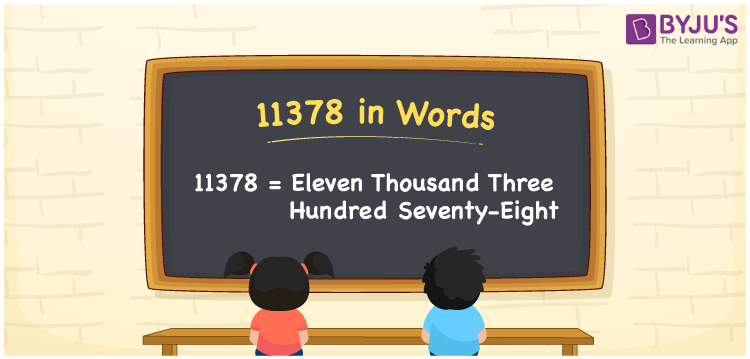# 11378 in Words

We can write 11378 in words as Eleven thousand three hundred seventy-eight. If you purchased a new swing chair that cost Rs. 11378, then you can say, “I purchased a new swing chair worth Eleven thousand three hundred seventy-eight rupees”. Let’s learn how to convert the number 11378 into word form with the help of a place value chart.

 11378 in words Eleven thousand three hundred seventy-eight Eleven thousand three hundred seventy-eight in Numbers 11378

## 11378 in English Words

Generally, we write numbers in words using the English alphabet. So, we spell 11378 in English words as “Eleven thousand three hundred seventy-eight”.## How to Write 11378 in Words?

As we can see, 11378 is a five-digit number, we need to create a place value chart with five columns to convert this number into words.

 Ten thousands Thousands Hundreds Tens Ones 1 1 3 7 8

Here, ones = 8, tens = 7, hundreds = 3, thousands = 1, ten thousands = 1

By expanding the above digits, we get;

1 × Ten thousand + 1 × Thousand + 3 × Hundred + 7 × Ten + 8 × One

= 1 × 10000 + 1 × 1000 + 3 × 100 + 7 × 10 + 8 × 1

= 10000 + 1000 + 300 + 70 + 8

= Ten Thousand + One thousand + Three hundred + Seventy + Eight

= Eleven thousand three hundred seventy-eight

Therefore, 11378 in words = Eleven thousand three hundred seventy-eight.

11378 is a natural number that is the successor of 11377 and predecessor of 11379.

11378 in words – Eleven thousand three hundred seventy-eight

Is 11378 an odd number? – No

Is 11378 an even number? – Yes

Is 11378 a prime number? – No

Is 11378 a composite number? – Yes

Is 11378 a perfect square number? – No

Is 11378 a perfect cube number? – No

## Frequently Asked Questions on 11378 in Words

Q1

### How do you write 11378 in words?

We can write number 11378 in words as Eleven thousand three hundred seventy-eight.
Q2

### How to write Rs. 11378 in words on a cheque?

On a cheque, we generally express an amount of Rs. 11378 in words as “Eleven thousand three hundred seventy-eight rupees only”.
Q3

### Find the difference between Eleven thousand three hundred seventy-eight and Four thousand two hundred ninety-eight.

Eleven thousand three hundred seventy-eight = 11378 Four thousand two hundred ninety-eight = 4298 Difference = 11378 – 4298 = 7080